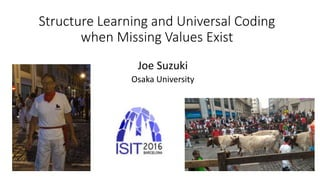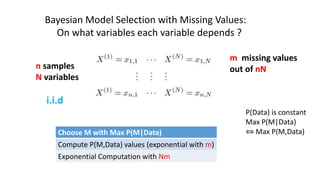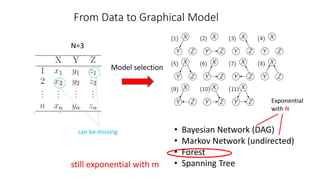Successfully reported this slideshow.

# 2016 7-13

0

Share×
1 of 18
1 of 18

# 2016 7-13

0

Share

Structure Learning and Universal Coding when Missing Values Exist
ISIT 2016, Barcelona, Spain, July 2016.

Structure Learning and Universal Coding when Missing Values Exist
ISIT 2016, Barcelona, Spain, July 2016.

## More Related Content

### Related Books

Free with a 14 day trial from Scribd

See all

### Related Audiobooks

Free with a 14 day trial from Scribd

See all

### 2016 7-13

1. 1. Structure Learning and Universal Coding when Missing Values Exist Joe Suzuki Osaka University
2. 2. m missing values out of nNn samples N variables Bayesian Model Selection with Missing Values: On what variables each variable depends ? P(Data) is constant Max P(M|Data) ⇔ Max P(M,Data)Choose M with Max P(M|Data) Compute P(M,Data) values (exponential with m) Exponential Computation with Nm i.i.d
3. 3. From Data to Graphical Model N=3 can be missing • Bayesian Network (DAG) • Markov Network (undirected) • Forest • Spanning Tree Model selection Exponential with N still exponential with m
4. 4. Contributions If the true model is expressed by a forest, and even if some values in the data are missing, 1. a model M with the max P(M|Data) is obtained in O(N^2) 2. If missing occurs stationary ergodic, maximizing P(M|Data) does not imply consistent estimation of M 3. An Exact Universal coding reducndancy in a precise form In this talk, we prove those statements one by one.
5. 5. Chow-Liu (1968)
6. 6. Universal Measures
7. 7. From Data: Structure Learning (Suzuki 93, 12)
8. 8. Generalization: missing values are present
9. 9. Proof Sketch: expand the forest from root
10. 10. Definitions
11. 11. Universal coding of forest data with missing values We generalize into the case that missing values are stationary ergodic by introducing
12. 12. Conclusions If the true model is expressed by a forest, and even if some values in the data are missing, 1. a model M with the max P(M|Data) is obtained in O(N^2) 2. If missing occurs stationary ergodic, maximizing P(M|Data) does not imply consistent estimation of M 3. An Exact Universal coding reducndancy in a precise form Future work: more general class of graphical models

### Editor's Notes

• My name is Suzuki, Osaka University.

Today, I will talk about such a theme.
• We consider a model selection that chooses a model with the maximum
posterior probability given the data. What I mean by a model is dependency among variables.

If N variables exist, exponential computation with N is required in general

In particular, we consider the situation that some values are missing.

Because we consider a Bayesian strategy, for m missing values, the computation is exponential with m as well.

• We may consider several graphical models: Bayesian netwroks, Markov networks, forests, and spanning trees, etc.

However, for example, for BNs, the computation will be exponential with m.

In order to reduce computation, in this work, we assume that the dependency is expressed by a forest.
• Here are the contributions of this work.

We assume that the dependency is expressed by a forest, and that some values in the data is missing.

First, a modet that maximizes the posterior probability can be obtained by O(N^2)
Second, we show that if missing occurs stationary ergodic, maximizing the posterior probability does not mean that
a correct model may not be obtained even for large n.

Finally, an exact universal coding redundancy will be shown in a precice form.
• Next let me explain Chow-Liu algorithm that approximate an original distribution by a spanning tree.

In 1968, they prove that the following procedure minimizes the K-L divergence between the original and forest distributions:

We repeatedly connect vertexes as an edge that maximize the mutual information if no loop is generated.

In this case, because 1 and 2 gives the maximum mutual information, they are connected first. Then, we connect the four vertexes in this order but 2 and 3 are not to be connected.
• Next, this may be a basic, but let me explain what universal measures are.

The measure gives a universal coding length.

The construction of such measures is very popular.

For more than one variable, almost similar construction is possible.
• Using the notion of universality, we can consider learning a forest structure.

If we assume that the prior probability is uniform over the forests, the problem reduces to maximizing this quantity.

To this end, Chow-Liu Algorithm can be used. In each step, we choose a pair that maximizes J^n(I,j) instead of the true mutual information values because they are not available.

If we define I^n(I,j) and alpha(i) to be the empirical MI and the numver of variables that variable X^{(i)} takes,
J^n(I,j) can be expressed by like this.

• The quantity J^n(I,j) gives a mutual information estimation.

If we do experiments, the estimation is fairly correct. In fact, we can prove this statement for large n.
• The first result is as follows.

If no missing values are present, the total score and the quantity that should be maximized in each step are like these.

The new result is in the right and generalizes the non-missing case.
Those four quantities are replaced.

If no missing valus exist, the left and right coincide.

• Here are examples.

For the first three quanitites, only the samples that X^{(i)} and X^{(j)} are observed.

In this case, only the five pairs are used out of n=10.

For the last quanity, only the samples that X^{(i)} is observed.

In this case, only the seven pairs are used out of n=10.

• Here’s a skech.

We evaluate the Bayes score.

First, we choose a root arbitrarily and expand the forest one by one.

Suppose we have obtained the score of X^{(1)} through X^{(i)}, and that X^{(i)} and X^{(i+1)} are to be connected.

Then, the left and right are data of X^{(i)} and X^{(i+1}.

We find the left here is multiplication of the two quantities like these.
• Before going into the second result, let me do easy definitions.

If missing events are stationary ergodic, we can define those probabilities.

• We have already defined the quantity J^n(I,j). But, we also define another estimation K^n(I,j).

Only the difference is the denominators of the two.

If no missing values are present, they are the same.

Under this assumption, K^n(I,j) converges to the true mutual information.

In other words, for K^n(I,j), consistency is guaranteed while the posterior probability is not maximized.

If the missing values are at most finite, the forests generated by them are asymptotically the same.

Proposition 2 concerns about independent testing. Both can detect independence as the sample size n grows.
• Finally, we consider universal coding of forest data with missing values.

If no missing values exist, the entropy can be expressed by
• Here are the contributions of this work.

We assume that the dependency is expressed by a forest, and that some values in the data is missing.

First, a modet that maximizes the posterior probability can be obtained by O(N^2)
Second, we show that if missing occurs stationary ergodic, maximizing the posterior probability does not mean that
a correct model may not be obtained even for large n.

Finally, an exact universal coding redundancy will be shown in a precice form.